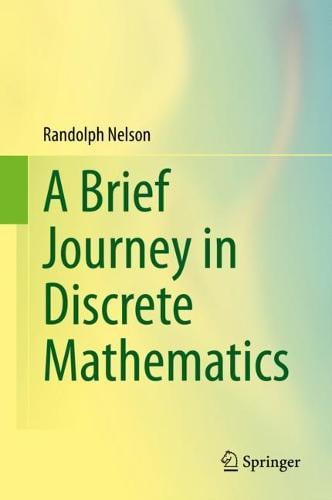•# A Brief Journey in Discrete Mathematics (Hardback)

(author)
£69.99
Hardback 142 Pages / Published: 30/04/2020
• Coming soon

Awaiting publication

The goal of this book is to showcase the beauty of mathematics as revealed in nine topics of discrete mathematics. In each chapter, properties are explored through a series of straightforward questions that terminate with results that lie at the doorstep of a field of study. Each step along the way is elementary and requires only algebraic manipulation. This frames the wonder of mathematics and highlights the complex world that lies behind a series of simple, mathematical, deductions.

Topics addressed include combinatorics, unifying properties of symmetric functions, the Golden ratio as it leads to k-bonacci numbers, non-intuitive and surprising results found in a simple coin tossing game, the playful, trick question aspect of modular systems, exploration of basic properties of prime numbers and derivations of bewildering results that arise from approximating irrational numbers as continued fraction expansions. The Appendix contains the basic tools of mathematics that are used in the text along with a numerous list of identities that are derived in the body of the book.

The mathematics in the book is derived from first principles. On only one occasion does it rely on a result not derived within the text. Since the book does not require calculus or advanced techniques, it should be accessible to advanced high school students and undergraduates in math or computer science. Senior mathematicians might be unfamiliar with some of the topics addressed in its pages or find interest in the book's unified approach to discrete math.

Publisher: Springer Nature Switzerland AG
ISBN: 9783030378608
Number of pages: 142
Dimensions: 235 x 155 mm
Edition: 1st ed. 2020